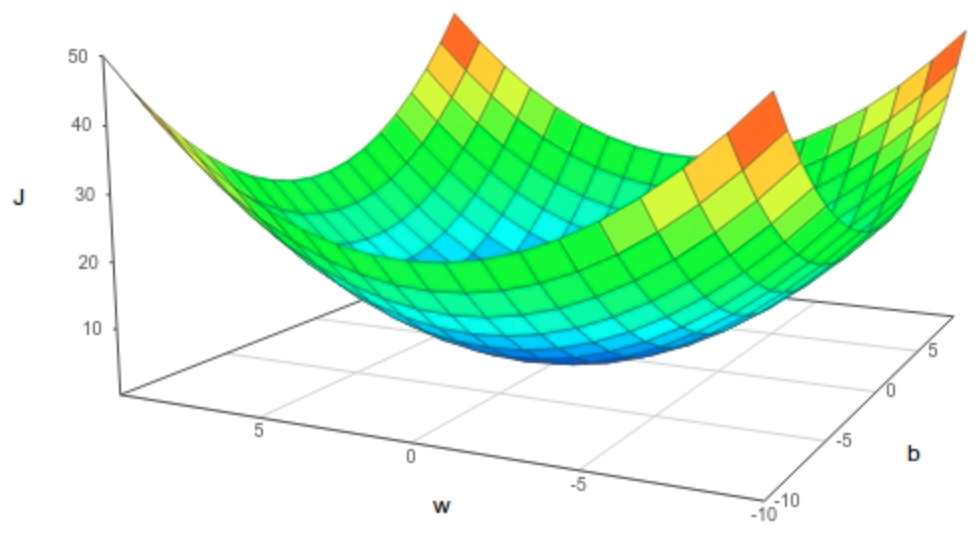# Neural Networks and Deep Learning W2— Shallow NN

Original article was published by Ozgur Guler on Deep Learning on Medium# Neural Networks and Deep Learning W2— Shallow NN

In this post, I will summarise the steps required to create a simple binary classification model through a single stage NN with sigmoid function as its activation function. It is amazing how such a simple model is effective in complex classification tasks!

For a binary classification problem, with a single layer Neural Network, input is processed with the following mathematical functions.

z = wT . x + b where w is the set of weights for linear processing (weighted sum of inputs) and b is a scalar.

a (activation) = y^ (our prediction) = σ (wT.x +b)

where σ (z) = 1/(1+exp(-z)).

For the simple binary classification, we are looking for the values of w and b that will minimise our prediction error. (e.g. we might be trying to predict weather a certain image is a cat image.)

This two step processing, weighted sum of inputs -the linear step- and the sigmoid of the output -non-linear step- is called the “forward propagation” phase of NN processing.

We define the following convex loss function that is well suited for convex optimisation (especially when compared to using standart mean squared error loss function)…

L (y^-y)= -(y log(y^) + (1-y)(log(1-y^)

Loss function will give us our prediction error for a single data sample.

Cost function (J) is the average of all individual losses from across your entire training set and is defined as…

J (w,b) = -1/m * Σ(i=1:m) (y[i] log(y^[i]) + (1-y[i])(log(1-y^[i])

Cost function J gives us the prediction error of our NN, for a specific w,b pair. This is the function we will be trying to minimise wrt to the weights (w) and the scalar b and with “Gradient Descent”

Gradient Descent is an iterative process where we are climbing down at the steepest downhill direction of the convex cost function (highest gradient) trying to reach the global optima — the lowest point in the cost function curve.

To do that we first calculate the derivative (gradient) of the cost function wrt w and b for each data point, then update w and b with their corresponding gradients for each iteration of our descent. This is called the “back propagation” phase of our NN processing.

w:=w-α. dJ(w) / d(w) , b:= w-α. dJ(b) / d(b) where alpha is our learning rate. (Learning rate determines the size of our steps while we are climbing down the convex cost curve.)

If we execute the partial derivatives with respect to w and b, we get the following…(Please refer to any “Math for Deep Learning” text for the derivations of db, dw, dZ.)

∂J/∂b = 1/m * X (A-Y).T

∂J/∂w = 1/m * Σ(i=1:m)(a[i]-y[i])

To execute the forward propagation step for m samples, we can use numpy vectorisation and leverage python broadcasting to avoid using an explicit for loop which is required to avoid the computational cost of the for loop. This is the power of numpy letting us achieve the matrix operation efficiently. In numpy this is done with np.dot(x,y) function.

Z = np.dot(w.T, X) +b, then write a sigmoid function and reach A.

A = σ(Z)

dZ = A -Y (this comes from the chain derivative rule )

dw = 1/m * np.dot(X, (A-Y).T)

db = 1/m * np.sum(A-Y)

w:=w-α. dJ(w) / d(w)

b:= w-α. dJ(b) / d(b)

With the above we don’t need a for loop for a single iteration of gradient descent by executing the forward propagation and the backward propagations. However if we would like to continue the optimisation we will need to iterate using a for loop over the rest of the samples.

For optimization we can define a function which will do the following…

for [number of iterations]

• forward propagate (calculate A and derive dw and db with a dedicated “propagate” function)
• back propagate- update w and b using dw and db

After optimization (n iterations of the optimization function) we will have optimal values for w and b for minimising the model cost.

Then finally with a predict function we can calculate our prediction y^, with

A = sigmoid(np.dot(w.T, X)+ b), and e.g. if the result is >0.5, we can predict a “YES”.

This completes the description of building a simple deep learning model for binary classification.